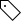QS世界大学排行出炉 中国41所大学入围500强

3所大陆大学综合实力进入全球50强，分别是清华大学第24名、北京大学第39名、复旦大学第43名。清华大学再创新高，位列全球第24名，这是自2006年后清华第二次将中国大陆带入世界25强大学。北大全球39名，上升2名。复旦大学上升8个名次至第43位，成为中国第三所进入世界前五十的大学。上海交大上升9位全球61。

 2016/2017年QS世界大学排行出炉 中国41所大学入围前500强 2016 2015 大学名称 地区 学术声誉 雇主声誉 教师学生比 教职论文引用数 国际教师比 国际学生比 总分 排名 排名 排名 排名 排名 排名 排名 排名 排名 24= 25 清华大学 大陆 28 22 95 81 327 401+ 86.0 27 30= 香港大学 香港 29 50 103 261 20 32 85.4 36 28 香港科技大学 香港 55 69 290 51 14 41 81.8 39 41 北京大学 大陆 14 14 176 139 287 289 81.3 43 51= 复旦大学 大陆 56 41 185 98 230 308 79.4 44 51= 香港中文大学 香港 49 86 182 205 54 147 78.1 55 57 香港城市大学 香港 131 277 106 42 17 46 75.4 61 70= 上海交通大学 大陆 65 38 346 78 211 401+ 72.2 68= 70= 台湾大学 台湾 38 121 357 74 401+ 401+ 70.2 104= 113= 中国科学技术大学 大陆 137 235 211 21 401+ 401+ 62.4 110 110= 浙江大学 大陆 101 91 401+ 45 401+ 401+ 61.6 111 116 香港理工大学 香港 133 214 279 167 45 139 61.4 115= 130= 南京大学 大陆 114 173 401+ 62 262 401+ 59.6 151 155 台湾清华大学 台湾 161 294 401+ 15 385 401+ 53.9 174 182= 台湾交通大学 台湾 311 401+ 213 58 354 256 50.1 241= 224 台湾成功大学 台湾 232 358 329 179 401+ 401+ 42.0 243 260 台湾科技大学 台湾 315 401+ 146 267 401+ 401+ 41.7 257 232 北京师范大学 大陆 187 365 401+ 301 337 401+ 40.4 275 273= 武汉大学 大陆 225 252 401+ 306 284 401+ 38.2 278= 291 哈尔滨工业大学 大陆 352 401+ 261 140 401+ 401+ 37.9 278= 281 香港浸会大学 香港 401+ 401+ 358 225 57 100 37.9 297= 307 中山大学 大陆 241 321 401+ 230 343 401+ 37.0 308= 338= 台湾阳明大学 台湾 401+ 401+ 33 309 401+ 401+ 36.2 310= 376= 台湾师范大学 台湾 266 401+ 214 401+ 401+ 156 36.0 315= 277= 南开大学 大陆 284 401+ 401+ 119 401+ 401+ 35.7 315= 345= 同济大学 大陆 285 399 401+ 313 222 401+ 35.7 318 331= 西安交通大学 大陆 308 401+ 266 314 401+ 401+ 35.6 389 401-410 北京理工大学 大陆 310 383 401+ 329 401+ 401+ 30.8 395= 379= 台湾中山大学 台湾 388 401+ 401+ 172 401+ 401+ 30.5 401-410 421-430 台北医学大学 台湾 - - 144 401+ 401+ 401+ 29.9 411-420 397= 台湾中央大学 台湾 - - 398 284 401+ 401+ 28.9 421-430 441-450 中国人民大学 大陆 - - 332 401+ 401+ 401+ 28.8 431-440 381= 北京航空航天大学 大陆 - - 401+ 274 401+ 401+ 28.2 441-450 441-450 华中科技大学 大陆 - - 401+ 244 401+ 401+ 27.4 441-450 461-470 长庚大学 台湾 - - 158 229 401+ 401+ 27.8 451-460 411-420 上海大学 大陆 - - 401+ 401+ 401+ 401+ 27.3 451-460 401-410 厦门大学 大陆 - - 401+ 290 401+ 401+ 27.0 471-480 471-480 华东理工大学 大陆 - - 401+ 85 401+ 401+ 26.1 481-490 大连理工大学 大陆 - - 401+ 108 401+ 401+ 25.6 481-490 421-430 天津大学 大陆 - - 401+ 253 401+ 401+ 25.7 491-500 451-460 吉林大学 大陆 - - 328 401+ 401+ 401+ 25.3
(责编：孝媛、汤龙)

推荐阅读每日头条